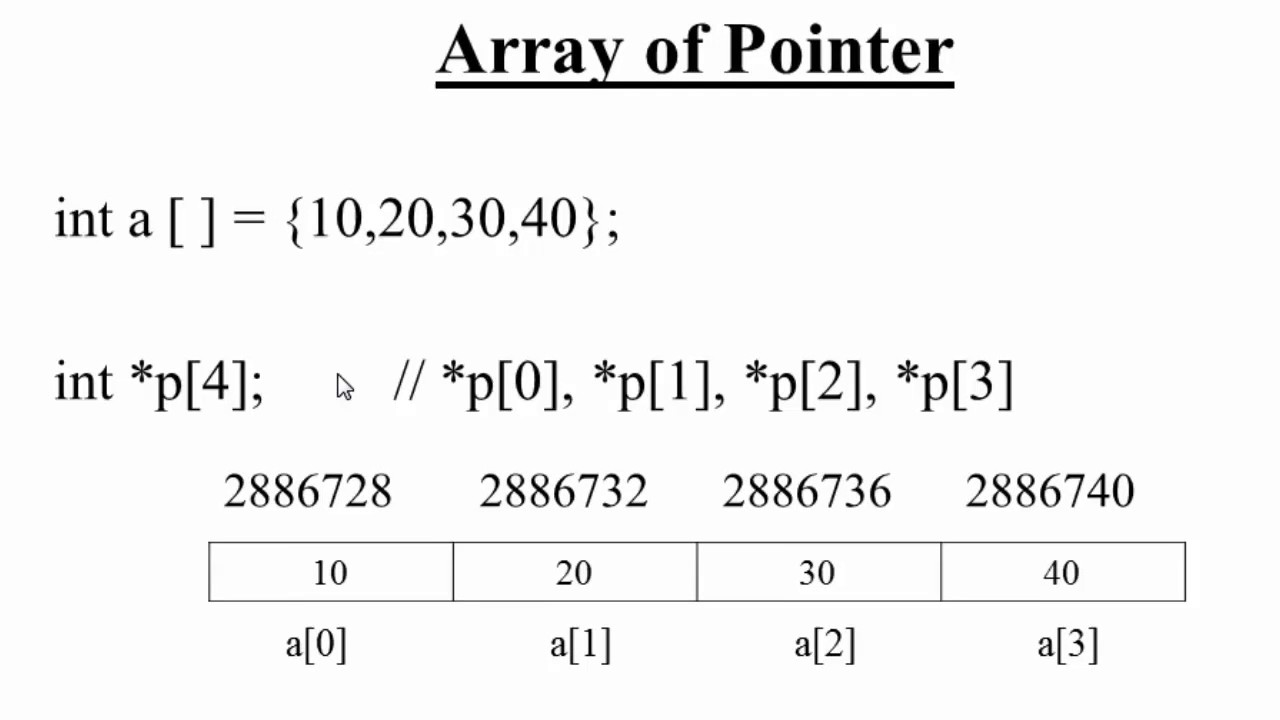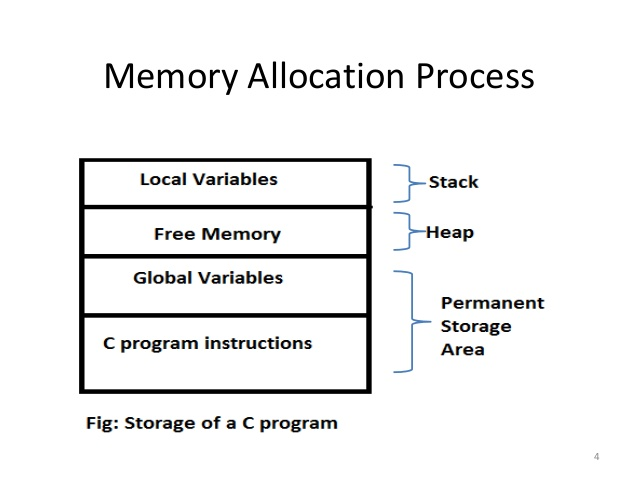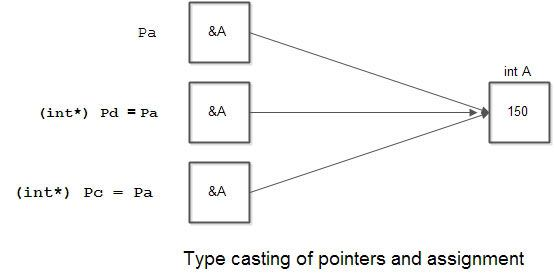×

Search anything:

# Understand Pointers in C in depth

#### Software Engineering C ProgrammingIndian Technical Authorship Contest starts on 1st July 2023. Stay tuned.

Reading time: 30 minutes | Coding time: 10 minutes

Pointers, as the name suggests, means pointing. In, computer programming terminology a pointer is a type of object that stores the address of a particular stored in a Computer system. A pointer references a location in memory and obtains the value stored at a location is known as dereferencing the pointer. The content allows us to get the value stored at an address held by a pointer.

## Syntax

``````datatype *var_name;
``````

As variables require declaration so does the declaration of a pointer so it can be utilized in the code. It just requires a sample * before the variable (eg. a, b, c etc.) and a data type (int, char, float).

# What exactly a pointer does?

Pointer has a simple mechanism and stores the addresses of various variables. It is very useful for passing a parameter, which is commonly known as pass by an address which will be covered later in this blog.

# Initializing pointer

After the declaration of the pointer here comes the next step called initializing. Initializing the pointer follows the same old procedural methods which are used for data types. To get the address of the variable, & operator (ampersand) is used before the variable whose address is to determined.
Note: You should avoid not to uninitialized pointers as it may give some anomalous results

# What are the types of pointers?

Pointers are of three types mainly called null pointer, Void pointer and wild pointer. Let's find more about each of them in detail.

• Null pointer: A null pointer is nothing but a command used to direct to an empty location in a computer system. It doesn't point to any variable or function and also used to denote the ending of memory search.
``````#include <stdio.h>
int main()
{
// Null Pointer
int *ptr = NULL;

printf("The value of ptr is %p", ptr);
return 0;
}
``````
• Void Pointer: It is a form of pointer which is used to point another variable of any data type. So, it can store the address of a variable of any data type.
``````int main()
{
int x = 4;
float y = 5.5;
void *ptr;
ptr = &x;
printf("Integer variable is = %d", *( (int*) ptr) );
ptr = &y;
printf("\nFloat variable is= %f", *( (float*) ptr) );
return 0;
}
``````
• Wild Pointer: The pointers which aren't initialized are called wild pointers. They are called wild because of the point to any arbitrary memory location which can cause a program to misbehave or crash eventually.
``````int main()
{
int *p;  /* wild pointer */
int x = 10;
p = &x;
return 0;
}
``````
• Dangling pointer: A dangling pointer is a pointer that doesn't point to a valid object and causes a program to crash.
``````#include<stdio.h>
int *fun()
{
static int x = 5;
return &x;
}
int main()
{
int *p = fun();
fflush(stdin);
printf("%d",*p);
}
``````

# Accessing the pointer

Pointers can be accessed in two ways, one is direct and other is indirect accessing.

• Direct access: Direct access can be done easily by using the variable name directly.
• Indirect access: Indirect access can be done easily by using a pointer to a variable.

# Operations on Pointers:

Some arithmetic operations can be applied on pointers. These include incrementing, decrementing, the addition of integer on a pointer and subtraction of a pointer from an integer.

### Now let's get straight to the concept of poiters and arrays

If you are not familiar with the concepts of arrays then it's not that tough. It is a sort of a structure (data) which contains a group of elements. The data used here are of the same type like int and char. These have a unique benefit of storing the elements in a sequential form that help us to locate the data we need to carry on with the rest of the application.How values are stored in an array and how we can use it to find the address at which it is stored.

# Initialzing the Array

The indexing is defined in terms of a pointer it self as the arrays are a subject of pointers. The syntax used for accessing arrays is the same as that to dereference the pointer. An array array is declared and can be used in the following manner:

``````int array;
int *ptr1 = array;
ptr1 = 1;
*(array + 1) = 2;
*(1 + array) = 2;
array = 4;
``````

This allocates a block of total 10 integers which serves as a pointer to the block. Pointers points the dynamically allocated memory from malloc which returns a block of memory of no less than the size that can be used as an array.

Values of the arrays can be declated as this:
int array = {2, 4, 3, 1, 5};

When we pass a pointer to a function by its address, the pointer's value gets copied from the argument to the function's parameter (int, void). This means it is passed by value. If you change the function parameter's value, you are only changing a copy.

``````void passByValue(int n) {
a = 5;
}
*b = 10;
}

int main(void) {
int c = 10;
passbyValue(c);
return 0;
}
``````

# Dynamic memory allocation (DMA)

Since resources are very limited. Our basic aim is to thrive the basic information in a memory. Through this, the concept of DMA or Dynamic memory allocation came. It refers to managing a system's memory during runtime i.e, memory should be allocated to the variables that were created and brought to existence. DMA is performed via a group four functions named malloc(), calloc(), realloc(), and free().# Type and casting Pointers

As we have discussed earlier that pointer values can be assigned to the other pointers of the same data type. However, pointers can also be typecast from one type to another type. Suppose, we have had different data formats such as a is an int type, b is char type and c is a float type. Hence, Pa is a pointer to an int type, Pb is a pointer to char type and Pc is a pointer to float type.

Example code:

``````int a= 150, *Pa;
Pa = &a;
char b, *Pb;
float c, *Pc,
``````

This code will give an error for Pb=Pc. Hence, the only method to resolve this is typecasting it.

Hence, when we change

``````Pb= (int*)Pd;
Pb = Pa;
(int*)Pc = pa;
``````

Code works perfectly fine. Hence, type casting solves various errors and After the execution of the code all the three-pointers, i.e., Pa, Pd, and Pc, points to the value 150.The above code shows how typecasting is done.

## Let's find some other pointers which are not known:

• Autorelative pointer: An auto relative pointer is a pointer whose value is an offset from the address of the pointer itself.
• Based Pointer: A based pointer is a pointer whose value is an offset from the value of other pointers.

These pointers are not that familiar to many as these are hardly encountered. Hope, now you are familiar with these as well.

## Use your brain and find the output:

``````include<iostream.h>
void fun(int x)
{
x = 30;
}

int main()
{
int y = 20;
fun(y);
cout<<"%d"<<y;
return 0;
}
``````

## What will be the output for the given code?

20
30
Runtime error
Compiler Error
Parameters are always passed by value in C++. Therefore, in the above code, value of y is not modified using the function fun().#### Shobhit Sinha

Sophomore at Thapar University | Intern at OpenGenus